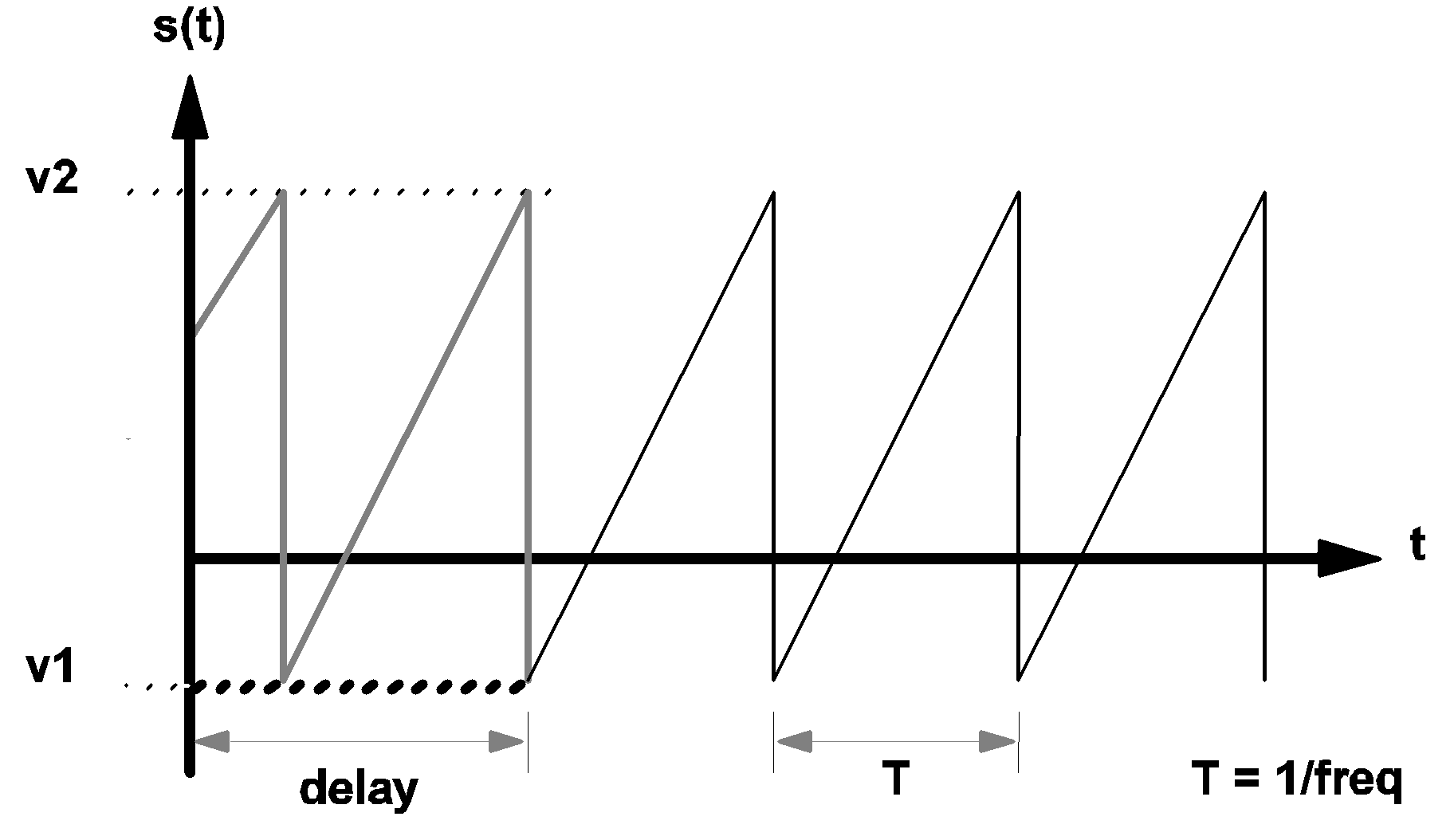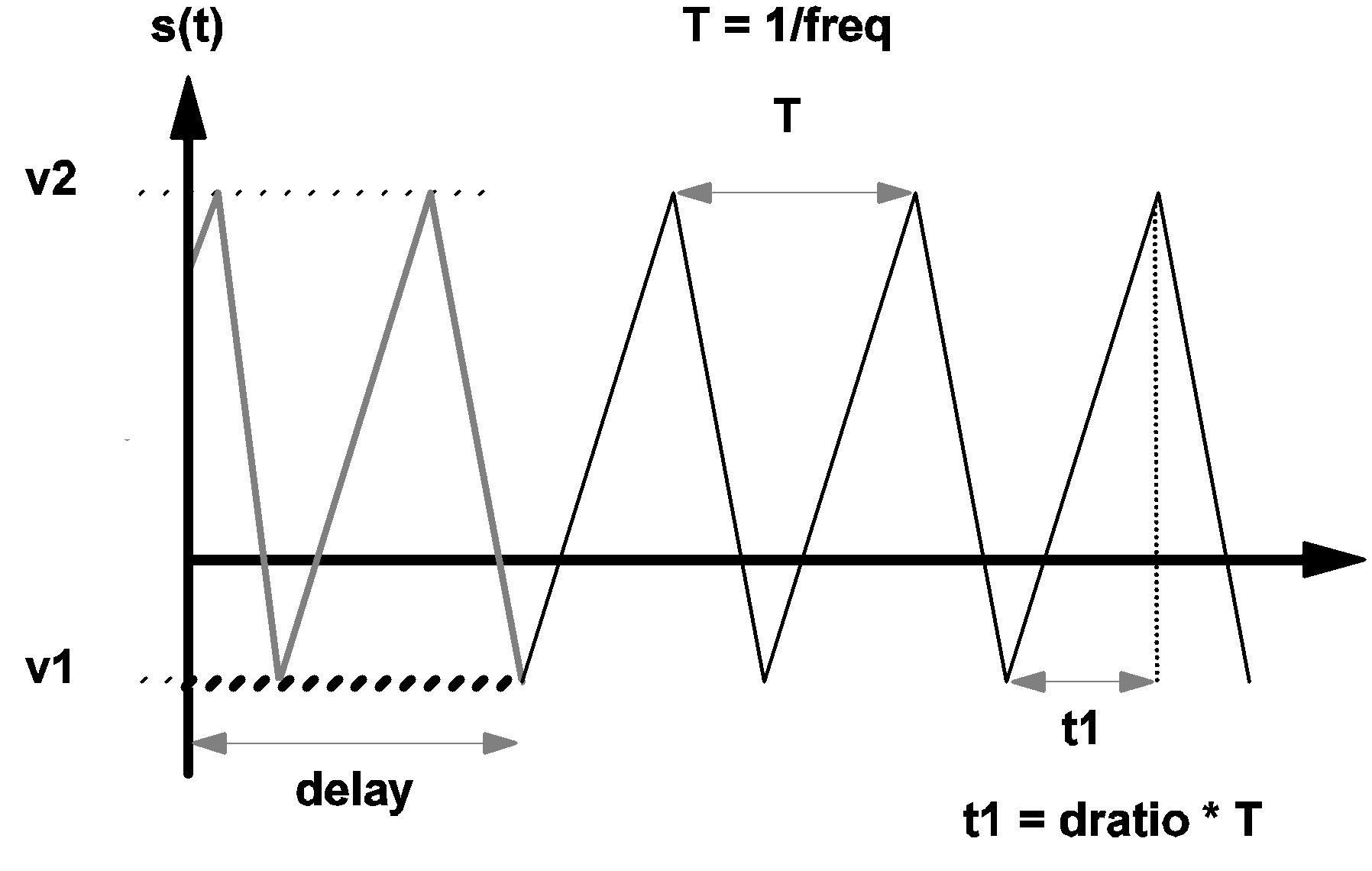# SIMPLIS Device Types

In this topic:

## Linear Resistors

The format for a linear resistor is:

Rname  n+  n-  value
where
 R is the one-character element keyword "R" for linear resistors name is the individual name of the device n+ is the name of the positive node, and is a nonnegative integer n- is the name of the negative node, and is a nonnegative integer value is a floating-point number assigned as the value of the resistance (in ohms). This value can be positive, zero, or negative

## Linear Inductors and Capacitors

The formats for a linear inductor and a linear capacitor are:

Lname  n+  n-  value  IC=init_cond
Cname  n+  n-  value  IC=init_cond
where
 L is the one-character element keyword "L" for linear inductors C is the one-character element keyword "C" for linear capacitor name is the individual name of the device n+ is the name of the positive node, and is a nonnegative integer n- is the name of the negative node, and is a nonnegative integer value is a floating-point number assigned as the value of the inductance (in henries) for an inductor or the value of the capacitance (in farads) for a capacitor. The value can be positive or negative, but not zero IC= is the three-character keyword "IC=" init_cond is a floating-point number assigned as the value of initial condition. It is the initial current (in amperes) for an inductor or the initial voltage (in volts) for a capacitor

## Independent Voltage and Current Sources

### DC Sources

The formats for the dc sources are:

Vname  n+  n-  DC  value
Iname  n+  n-  DC  value
where
 V is the one-character element keyword "V" for independent voltage sources I is the one-character element keyword "I" for independent current sources name is the individual name of the device n+ is the name of the positive node, and is a nonnegative integer n- is the name of the negative node, and is a nonnegative integer DC is the two-character keyword "DC" to signify that this is a DC source value is a floating-point number assigned as the source value. It is the voltage across the source element (in volts) for a dc voltage source or the current through the source (in amperes) for a dc current source

### Sawtooth Sources

The format for the independent sawtooth voltage source is:

Vname  n+  n-  SAW  V1=v1  V2=v2
+ FREQ=freq  DELAY=delay
+ OFF_UNTIL_DELAY={YES|NO}
+ IDLE_IN_POP=YES|NO
The format for the independent sawtooth current source is:
Iname  n+  n-  SAW  V1=v1  V2=v2
+ FREQ=freq   DELAY=delay
+ OFF_UNTIL_DELAY={YES|NO}
+ IDLE_IN_POP=YES|NO
where
 V is the one-character element keyword "V" for independent voltage sources I is the one-character element keyword "I" for independent current sources name is the individual name of the device n+ is the name of the positive node, and is a nonnegative integer n- is the name of the negative node, and is a nonnegative integer SAW is the three-character keyword "SAW" to signify that this is a sawtooth source V1= is the three-character keyword "V1=" representing the source value at the start of a normal cycle v1 is a floating-point number assigned as the value of V1 (in volts) for a voltage source and the value of V1 (in amperes) for a current source V2= is the three-character keyword "V2=" representing the source value at the end of a normal cycle v2 is a floating-point number assigned as the value of V2 (in volts) for a voltage source and the value of V2 (in amperes) for a current source FREQ= is the five-character keyword "FREQ=" freq is a positive floating-point number assigned as the frequency of this source (in hertz) DELAY= is the six-character keyword "DELAY=" delay is a floating-point number assigned as the value of DELAY (in seconds) OFF_UNTIL_DELAY= is the sixteen-character keyword "OFF_UNTIL_DELAY=" YES is the three-character keyword "YES" NO is the two-character keyword "NO" IDLE_IN_POP= may have values YES or NO. Default is NO. If YES, the source will be inactive during POP and AC analyses. Inactive means that the source will hold its t=0 value throughout the analysis. If NO the source will behave normally during POP and AC analyses
The plus characters shown in the format definition are not necessary if carriage returns are not used in the statement. The plus characters and the carriage returns have been added to break the statements over different lines to make them easier to read. Using the function s(t) to represent the voltage across the voltage source or the current through the current source, the value of the source function s(t) in the diagram below for $t > \textit{delay}$ is defined as:

$s(t)=v1+[(v2-v1)(t-\textit{delay})]/T$ for $\textit{delay} < t < (\textit{delay} +T)$

and:

$s(t)=s(t-T)$ for $(\textit{delay} +T) < t$

where

 T=1/(freq) is the period of the waveform
The source function s(t) for $t < \textit{delay}$ is defined as follows

$s(t) = s(t+T)$ for $0 < t < \textit{delay}$ and OFF_UNTIL_DELAY=NO

and

$s(t)=v1$ for $0 < t < \textit{delay}$ and OFF_UNTIL_DELAY=YES

Whether the delay is positive or negative, the time-domain transient analysis performed by SIMPLIS always starts with the time variable set equal to 0.0.

The diagram below shows the waveforms of a sawtooth source. For t < delay and OFF_UNTIL_DELAY=YES, the waveform s(t) is shown in bold dashed line. For t < delay and OFF_UNTIL_DELAY=NO, the waveform s(t) is shown in heavy gray line.3.2 Waveform s(t) of a sawtooth source

## Triangular Sources

The formats for independent triangular voltage and current sources are:

Vname  n+  n-  TRI  V1=v1  V2=v2
+ FREQ=freq  DRATIO=dratio  DELAY=delay
+ OFF_UNTIL_DELAY={YES|NO} IDLE_IN_POP=YES|NO

and

Iname  n+  n-  TRI  V1=v1  V2=v2
+ FREQ=freq  DRATIO=dratio  DELAY=delay
+ OFF_UNTIL_DELAY={YES|NO} IDLE_IN_POP=YES|NO
where
 V is the one-character element keyword "V" for independent voltage sources I is the one-character element keyword "I" for independent current sources name is the individual name of the device n+ is the name of the positive node, and is a nonnegative integer n- is the name of the negative node, and is a nonnegative integer TRI is the three-character keyword "TRI" to signify that this is a triangular source V1= is the three-character keyword "V1=" representing the source at the start of a normal cycle v1 is a floating-point number assigned as the value of V1 (in volts) for a voltage source or the value of V1 (in amperes) for a current source V2= is the three-character keyword "V2=" representing the source at the end of a normal cycle v2 is a floating-point number assigned as the value of V2 (in volts) for a voltage source or the value of V2 (in amperes) for a current source FREQ= is the five-character keyword "FREQ=" freq is a positive floating-point number assigned as the frequency of this source (in hertz) DRATIO= is the seven-character keyword "DRATIO=" dratio is a dimensionless floating-point number between 0.0 and 1.0, exclusively, assigned as the value of DRATIO DELAY= is the six-character keyword "DELAY=" delay is a floating-point number assigned as the value of DELAY (in seconds) OFF_UNTIL_DELAY= is the sixteen-character keyword "OFF_UNTIL_DELAY=" YES is the three-character keyword "YES" NO is the two-character keyword "NO" IDLE_IN_POP= may have values YES or NO. Default is NO. If YES, the source will be inactive during POP and AC analyses. Inactive means that the source will hold its t=0 value throughout the analysis. If NO the source will behave normally during POP and AC analyses
The source function s(t) for t > delay is defined as follows:

$s(t)=v1+[(v2-v1)(t-\textit{delay})]/t1$

for delay < t < (delay + t1)

$s(t)=v2+[(v1-v2)(t-\textit{delay}-t1)]/(T-t1)$ for $(\text{delay} + t1) < t < (\text{delay} + T)$

and

$s(t)=s(t-T)$ for $(\textit{delay} + T) < t$

where

 T=1/(freq) is defined as the period of the waveform t1=DRATIO*T is the duration in a period of the waveform where the source value is moving from the value of v1 to the value of v2.
The source function s(t) for t < delay is defined as follows:

$s(t)=s(t+T)$ for $0 < t < \textit{delay}$ and OFF_UNTIL_DELAY=NO

and

$s(t)=v1 for 0 < t < \textit{delay} \text{ and OFF_UNTIL_DELAY=YES.}$ Again, whether the delay is positive or negative, the time-domain transient analysis performed by the simulation always starts with the time variable set equal to 0.0. The diagram below shows the waveform, s(t), of a typical triangular source. For t < delay and OFF_UNTIL_DELAY=YES, the waveform s(t) is shown in bold dashed line. For t < delay and OFF_UNTIL_DELAY=NO, the waveform s(t) is shown in heavy gray line.3.3 Waveform s(t) of a triangular source

## Square Wave Sources

The formats for independent square-wave voltage and current sources are:

Vname  n+  n-  SQU  V1=v1  V2=v2  FREQ=freq
+ DELAY=delay  OFF_UNTIL_DELAY={YES|NO}
+ [IDLE_IN_POP=YES|NO]

and

Iname  n+  n-  SQU  V1=v1  V2=v2  FREQ=freq
+ DELAY=delay  OFF_UNTIL_DELAY={YES|NO}
+ [IDLE_IN_POP=YES|NO]
where
 V is the one-character element keyword "V" for independent voltage sources I is the one-character element keyword "I" for independent current sources name is the individual name of the device n+ is the name of the positive node, and is a nonnegative integer n- is the name of the negative node, and is a nonnegative integer SQU is the three-character keyword "SQU" to signify that this is a square source V1= is the three-character keyword "V1=" representing the source at the start of a normal cycle v1 is a floating-point number assigned as the value of V1 (in volts) for a voltage source and the value of V1 (in amperes) for a current source V2= is the three-character keyword "V2=" representing the source at the end of a normal cycle v2 is a floating-point number assigned as the value of V2 (in volts) for a voltage source and the value of V2 (in amperes) for a current source FREQ= is the five-character keyword "FREQ=" freq is a positive floating-point number assigned as the frequency of this source (in hertz) DELAY= is the six-character keyword "DELAY=" delay is a floating-point number expressing DELAY (in seconds) OFF_UNTIL_DELAY= is the sixteen-character keyword "OFF_UNTIL_DELAY=" YES is the three-character keyword "YES" NO is the two-character keyword "NO" IDLE_IN_POP= may have values YES or NO. Default is NO. If YES, the source will be inactive during POP and AC analyses. Inactive means that the source will hold its t=0 value throughout the analysis. If NO the source will behave normally during POP and AC analyses
The source function s(t) for $t > \textit{delay}$ is defined as follows:
 $s(t)=v2$ for $\textit{delay} < t < (\textit{delay} + T/2)$: $s(t)=v1$ for $(\textit{delay} + T/2) < t < (\textit{delay} + T)$: $s(t)=s(t-T)$ for $(\textit{delay} + T) < t$:

The source function s(t) for $t < \textit{delay}$ is defined as follows

## Subcircuit Calls / Instantiation

The format for a subcircuit call is:

Xname  n1  n2 ...  nn  sname
where
 X is the one-character element keyword "X" for the subcircuit call/instantiation name is the individual name of the device n1 is a nonnegative integer to represent the name of the first node of this device n2 is a nonnegative integer to represent the name of the second node of this device
nn is the nonnegative integer representing the nth node of this device, and sname is the name of a subcircuit definition compatible with this device. Through the use of the subcircuit feature, one can model an n-terminal physical device by building an n-terminal subcircuit made up of the simple basic devices outlined in this section. The subcircuit feature is further explained in See Subcircuit Definition.